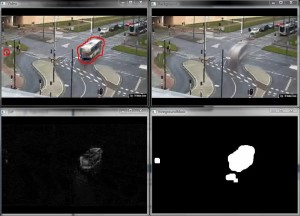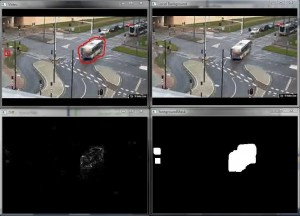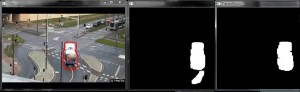Posted on

# Moving Vehicle DetectionThe goal of this project is to implement algorithm that segments foreground using OpenCV library. We assume that background is static, objects in foreground are moving and video is taken from static camera. We detect movingÂ  vehicles (foreground) with 2 methods.

## Background detection

First method is computing an average image from video frames.

1. Each frame is added into accumulator with a certain small weight (0.05 and smaller)
1. accumulateWeighted(frame, background, alpha);
2. at this point we have in Mat background actual backgorund image
2. To detect foreground we have to compute difference between current frame and current accumulated background image
1. absdiff(frame, background, diff);
2. in Mat diff is color image, we need to transform it into grayscale image
3. To detect relevant changes (more than given threshold) we use simple
1. thresholdthreshold(diff, foregroundMask, 20, 255, CV_THRESH_BINARY);
4. We can use morphological operations (dilatation, erosion) to expand foreground region

Each steps (1-4) are illustrated in next figures## Frames history

Second method is compare current frame with older frame. It is enough if the stack is 5-20 elements large- it depends on the speed of vehicles.

1. Add current frame into stack (if stack is full, first element is erased and the rest is shifted)
2. Compute difference between current frame and first element in the stack
1. first = framesHistory.first();
2. absdiff(frame, first, diff);
3. To detect relevant changes (more than given threshold) we use simple threshold
1. threshold(diff, foregroundMask, 20, 255, CV_THRESH_BINARY);
4. We can use morphological operations (dilatation, erosion) to expand foreground region

Each steps (1-4) are illustrated in next figures. LocalBackgound is the older frame (5 frames old)## Combination of methods

These 2 methods are combined to create more accurate output. In the next picture we can see that first way (computing average image) creates â€œtailsâ€ behind vehicles. Comparing with older frame doesnâ€™t create â€žtailsâ€œ.We use simple sum of binary masks

`Mat sumMask = mask1 & mask2`

## How to create more precise segmentation, future work

To create more precise vehicle segmentation we have to use another methods. Shadows of cars, lights of cars make these 2 methods to hard use. We can only segment region where could be a car, but for more precisely detection we have to use template matching (champfer matching), graph cut method. In this project we experimented with these other two, but these were too complex, so the time complexity was unacceptable to use in video.